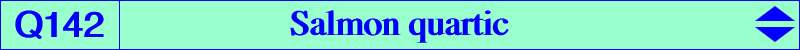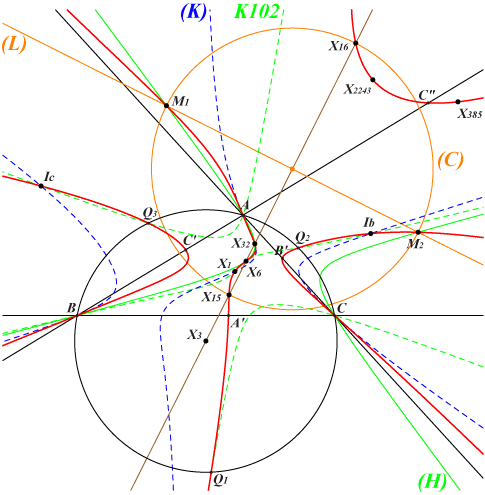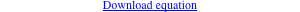∑ b^2 c^2 x^3 (c^4 y - b^4 z) = 0X(1), X(6), X(15), X(16), X(32), X(385), X(2243) excenters six feet of bisectors vertices of Grebe triangle other points belowLet M be a point and denote by K1, K2 the two associated cubics pK(X6, M) and pK(M, X6) respectively. These two cubics are anharmonically equivalent (see Salmon, Higher plane curves) if and only if M lies on the quartic Q142. The most remarkable examples are K128 = pK(X6, X385) and K739 = pK(X385, X6), each being the X(2)-Hirst transform of the other. *** The locus of P whose polar line in Q142 passes through X(32) is the cubic (K) = pK(X6, X76) = K1027 hence the tangents to Q142 at A, B, C, the in/excenters and obviously X(32) must pass through X(32). Note that A, B, C are points of inflexion on Q142. X(6) is also a point of inflexion with tangent passing through X(2) and X(385). Q142 meets the Lemoine axis at two imaginary points on the circumcircle (O) and two real points M1, M2 on the circum-conic (H) with perspector X(669), passing through X(6), X(32), etc. The circle (C) with diameter M1, M2 contains X(15), X(16) and is orthogonal to (O). The midpoint of M1, M2 is X(5027). M1, M2 also lie on the Feuerbach hyperbola of the tangential triangle, on the cubics K035 and K150 and are now X(18773), X(18774) in ETC. Q142 meets (O) again at three real points Q1, Q2, Q3 which are the vertices of the Grebe triangle, on the Grebe cubic K102 and many other cubics. See K644 and Table 57. *** The point X(6) above may be replaced with any point P = p : q : r and an analogous quartic Q(P) is obtained likewise with equation ∑ q r x^3 (r^2 y - q^2 z) = 0 or ∑ p^3 y z (r y^2 - q z^2) = 0. Q(P) passes through P, P^2, the square roots of P, the X(2)-Hirst inverse of P. Other properties similar to those of Q142 are easily found. *** Q142 is the locus of P such that X(32), P and P*/P are collinear. See Q002, locus property 9, for other similar curves.